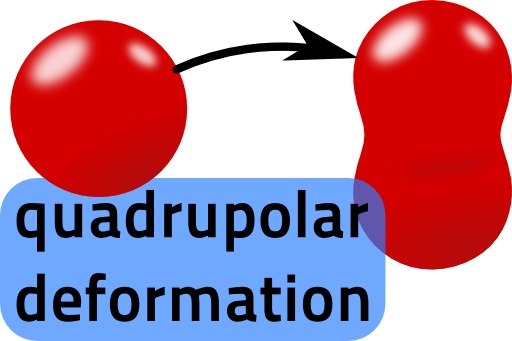## A Simple Model for Atomic Nuclei: The Deformed Sphere and its Multipole MomentsWe will calculate the first three multipole moments of a deformed charged sphere. Such a charge distribution is often used to model nuclei and understand their stability. The rotational symmetry of the problem will ease our computations significantly.

## Problem StatementCalculate the first three multipole moments of a slightly deformed sphere with homogeneous charge distribution $$\rho\left(\mathbf{r}\right)$$ as depicted on the right. Mathematically, the surface of the deformed sphere is paramtrized by $R\left(\theta,\varphi\right) = R_{0}\left(1+\xi P_{2}\left(\cos\theta\right)\right)\ ,$with the Legendre polynomial of second order, $$P_{2}\left(\cos\theta\right)=\left(3x^{2}-1\right)/2$$.

Assume that the deformation parameter $$\xi\ll1$$ and calculate $$\rho$$'s total charge, dipole moment and quadrupole moment approximately in leading order of $$\xi$$. Note that in rotational symmetry we have to calculate only one moment per order as discussed in "Multipole Moments in Rotational Symmetry".

We use the second order Legendre polynomial to achieve the deformation. This order is naturally linked to the quadrupole moment. So we could say that the deformation itself is of "quadrupolar nature". Shortly discuss if this kind of deformation implies that higher order multipole moments vanish.

## Background: From Electrostatics to Nuclear Physics - the Stability of Nuclei

Nowadays we are celebrating the proof of the Higgs boson, which was found using huge laboratories and enormous efforts to handle the enormous amounts of created data. These results are basically the proof of the standard model and physicists around the world are eager to find even more in-depth questions requiring even more sophisticated methods to verify. But of course these findings are at the end of a long journey of humans to understand the nature of the fundamental particles.When Robert Hofstadter, an American physicist (Nobel prize 1961), started to make huge contributions to the understanding of the structure of nuclei, it was widely believed that these tiny things did not have any inner structure. Knowledge has advanced and we can perform scattering experiments to reveal nuclei machinery. Their charge distribution can be approximated by a sharp boundary$R\left(\theta\right) = R_{0}\left(1+\xi_{2}P_{2}\left(\cos\theta\right)+\xi_{4}P_{4}\left(\cos\theta\right)\right)\ ,$very similar to the charge distribution we made ourself familiar with. On the right you can see contour plots of spheres with different kinds of deformation - no (blue), quadrupolar (magenta) and octupolar (yellow). The deformation parameters $$\xi_{i}$$ are in direct relation to the stability of a nucleus. If the quadrupole- and octadupole deformation parameters $$\xi_{1}$$ and $$\xi_{2}$$ are small, the nucleus is close to spherical symmetry. Such nuclei are extremely stable, examples are $$^{4}\text{He}$$  and $$^{208}\text{Pb}$$. Unstable elements with larger deformation parameters are the rare earths or the actinoides including $$^{238}\text{U}$$. The reason behind the stability is the binding energy. The higher it is, the more favorable is a symmetric shape. For smaller binging energies, repulsive forces inside the nucleus are considerable and thus lead to the described deformations. In the end, if one can measure the measurement of multipole moments thus gives a fundamental insight into their inner structure - electrostatics takes an important place to understand matter even in times of the Higgs boson!

Further reading: You may enjoy the MIT open courseware lectures on applied nuclear physics, link (a basic understanding of quantum mechanics will be necessary). Writtin in a more popular style is the freely available book “Nucleus - a trip in the heart of matter”.